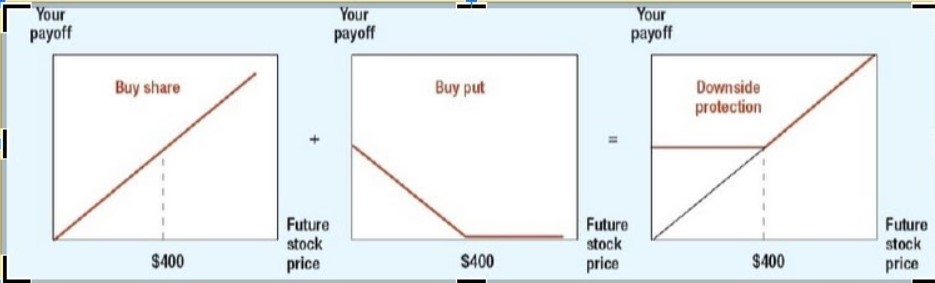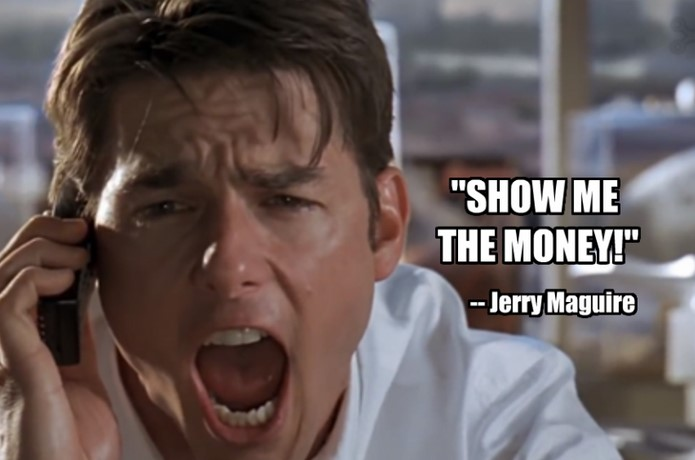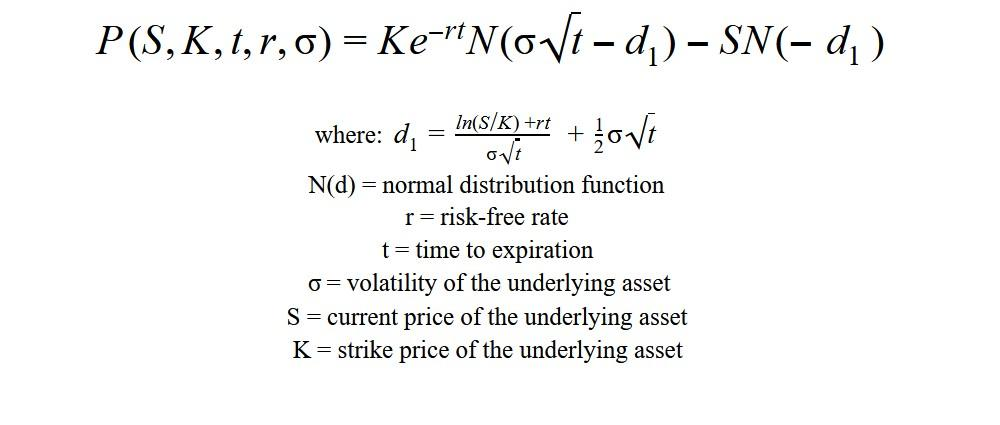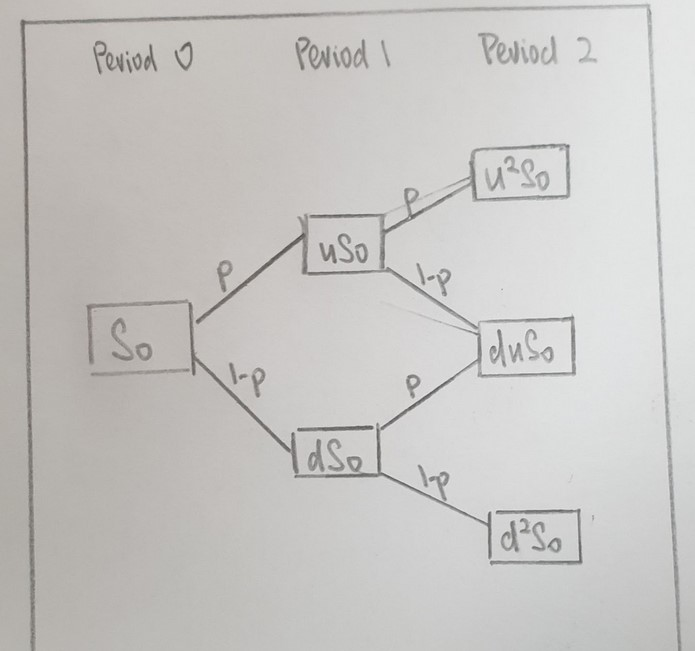# Put Option

What is a Put Option?

A Put Option (commonly referred to as put) is a derivative that allows the holder the right, but not the obligation, to sell an asset to the writer of the option at a predetermined strike price within or on the expiration date, depending on the nature of the option bought. The figure below illustrates the payoff on a put option in relation to the price of the underlying asset.Downside protection would be obtained from buying a put option for the share of a company as the gain from the put option during such a scenario would cancel out the loss from the decrease in the price of the share. (image assumes perfect capital markets)

There are two primary types of put options that are most common, and their differences are around what rights are given to the holder.

American Option:

• The holder is allowed to exercise the option as long as it is within (on or before) the expiration date

European Option:

• The holder is only allowed to exercise the option on the expiration date.

In practice, this is a huge difference and suggests that the American version is more flexible than a European version and therefore, commands a premium over the European Put (other things being equal).

## Puts "In-the-money" vs "Out-of-the-money"

A put with the price of the underlying asset below or on the predetermined strike price would be considered "in-the-money" and therefore possesses intrinsic value. More simply, this means that if the holder exercised the right to sell today, it would be worth something (the cash payoff on the option).

On the contrary, a put with the price of the underlying asset above the predetermined strike price would be considered "out-of-the-money." Simply put, this means that if the holder exercised the right to sell their share in the illustrated condition, they would make a loss as the predetermined strike price plus the extrinsic value of the put would be lesser than the share price itself. Therefore, a rational investor would sell the shares directly and not exercise the put when it is "out-of-the-money."

However, so long as there is still time before the expiry of the put, it still has some extrinsic value. Therefore, despite the fact that it may be "out-of-the-money" now, there are factors that would contribute to the put having extrinsic value. Some of the factors include the volatility of the underlying asset, which can be measured by vega.### In the Money - Put Option Example

Let's say investor A buys a put on Apple stock at \$170 per share that expires in 6 months. This can be expressed as follows:

Strike Price: \$170
Time to Expiry: 6 months
Underlying Asset: AAPL

Let's assume that Apple stock is currently trading below \$170. For this example, let's assume it is trading at \$160, then we would call this option "in-the-money'' because A has the right to sell something for \$170 that is only currently worth \$160. Since the shares can be bought in the open market for \$160 and sold to the put writer for \$170, it means a profit of \$10 for A.

### Out of the Money - Put Option Example

A put with the price of the underlying asset above the predetermined strike price would be considered "out-of-the-money" and only possesses extrinsic value.

Continuing the same example from above, let's say Investor A buys a put on Apple stock at \$170 per share that expires in 12 months. This can be expressed as follows:

Strike Price: \$170
Time to Expiry: 12 months
Underlying Asset: AAPL

Let's assume Apple stock is now above \$170. In this example, let's say it's trading at \$175. In this case, then we would consider this option to be "out-of-the-money" because A has the right to sell AAPL for \$170 that is currently worth \$175. However, as there is a significant amount of time before the expiry of the option, it still carries extrinsic value. This means that being "out-of-the-money" now is definitely a money-losing event.

Key point: However, even though this option may be "out-of-the-money", it doesn't mean it still doesn't have value.

Since the option still has time before it expires, it is still providing downside protection for Investor A. Therefore, for volatile stocks like Broadcom (or any underlying volatile asset) with many months (in this example) before expiry, these options may actually be worth quite a lot!

For a more detailed explanation, check out this video:

## Protective Put (Married Put)

The most common use case for put is during a risk-management strategy known as protective put (or married put). This is the purchase of the option as an insurance policy. This strategy is usually undertaken when an investor, holding a long position in the underlying asset, purchases an in-the-money option to protect against the decline of the price of the underlying asset.

### Why Buy a Protective Put?

• First, the underlying asset that the investor had purchased the protective put for may have had a huge increase in price and is likely to decline in value in the short run. The investor, upon knowing such information, would purchase a Protective Put to secure some of the profits they have earned over time.
• Second, new information (possibly negative) relating to the underlying asset that will affect the profitability or value of the underlying asset would increase the volatility of the underlying asset as investors would need time to figure out the new optimal valuation after taking into account the new information. An investor, upon knowing that there may be a downside, may be enticed to purchase a protective put to protect themself from the downside scenario.
• Third, an investor may purchase an asset that is new and does not have an underlying track record of its performance. They would simultaneously purchase a protective put that is distant in expiration date to protect their capital during the time period of their purchase to the expiration date of a decrease in the price of the asset.

An alternate strategy involving the put is a straddle. This strategy involves the simultaneous purchase of a call option and a put of the same predetermined strike price and expiration date. This strategy is typically implemented when an investor is convinced that the underlying asset of the option is prone to an increase in volatility, but has less conviction in which direction the pricing will move.*not inclusive of fees

An example of when a straddle trade might make sense was during the March 2020 stock market crash. In this case, when investors may take a while to form an educated opinion on the full impact of the COVID-19 crisis, Saudi-Russia oil price war, and the closing of borders by countries, a straddle trade would allow them to bet on volatility increasing without an opinion on whether prices will continue to drop or shoot back up with the announcement of stimulus packages (for example).

Many derivative traders, taking advantage of the high volatility reflected in the VIX index, employ it in their trading when there is no definitive conclusion if the stock markets have "bottomed" or will continue to decline.

## Value of Put Options: 5 Factors

In general, there are 5 factors that affect the value of options. They are the strike price, price of the underlying asset, time to expiration, interest rate, and volatility of the underlying asset respectively.

### Strike Price

The strike price is the predetermined price to sell the underlying asset. When there is an increase in the strike price of the put, its value increases. The rationale is that as the strike price increases, the higher the chances that the put is "in-the-money" and above the prevailing price of the underlying asset. This makes it more likely that the put has intrinsic value and/or commands a better premium, which pushes up its overall value.

### Price of the Underlying Asset

The price of the underlying asset affects the value of the put directly. When there is an increase in the price of the underlying asset, there is a decrease in the value of the put. This is because when there is an increase in the price of the underlying asset, there is a lower probability that the put is "in-the-money" and above the prevailing price of the underlying asset. This reduces its intrinsic value. The direct effect of change in the price of the underlying asset on the price of an option is measured through a factor that is known as delta.

### Time to Expiration

The time to expiration of the put affects its value directly. When the length of the expiration of the put is long, there is an increase in the extrinsic value of the put. This is because in this case, there is a long period of time for the price of the underlying asset to fall below the strike price of the put. This allows the option to command a higher premium, due to its increased extrinsic value. However, as the option nears its time to expiration, the extrinsic value of the put keeps decreasing. This is due to the effect of the time value of money and is called the time decay of an option, commonly measured through a factor called theta.

Further, it is not in all circumstances where a long time to expiration would increase the value of the put. For example, if the put purchased is a European option (recall earlier that European option could only be exercised at the expiry date) and the company is in financial distress, it is likely that the European put with a short date to expiration would have more value than the European put with a long date to expiration. This is due to the uncertainty of how long the financially distressed company could last which would bind the European option holder for a longer period of time.

### Interest Rate Environment

The prevailing risk-free interest rate directly affects the value of the put. When the interest rate is high, there is a decrease in the extrinsic value of the option. This is because when the interest rate is high, the interest forgone from purchasing the put rather than shorting the underlying asset and putting the cash from the short in an interest-bearing instrument is higher.

### Implied Volatility of the Underlying Asset

The implied volatility (IV) of the underlying asset affects the value of the put. When the IV of the underlying asset is high, there is an increase in the extrinsic value of the option. This is because when the IV of the underlying asset is high, more investors of the underlying asset would want to purchase insurance against a possible downturn of the underlying asset. This is because when there is a downturn, the decline in value would be larger. With more demand for the put, the value increases.

Further, to explain the concept of IV, it is only possible to estimate the future volatility of an underlying asset with credible information. Therefore, investors would use the historical volatility of the underlying asset to infer the future volatility of the underlying asset. This is what is commonly known as implied volatility (IV).

## Pricing a Put Option

There are many ways to price a put. We will be discussing the most common ways to price a put, namely the Black-Scholes-Merton Model and the Binomial Option Pricing Model.

### Black-Scholes Option Pricing Model

The Black-Scholes-Merton Model (more commonly known as Black-Scholes Option Pricing Model) won the 1997 Nobel Memorial Prize in Economic Sciences. It is applied in European options that do not pay dividends. In the event that the underlying asset pays dividends, an adjustment could still be made to fit it into the Black-Scholes model. The adjustment is as follow:

Sx = S - PV(Dividends)

where: Sx = adjusted underlying asset price
S = underlying asset price
PV(Dividends) = present value of all dividends to the expiration date of the option

Once the adjustment is made, it can be plugged into the Black-Scholes formula to derive the value of the European put. The formula of the Black-Scholes model to derive the value of a put is as follow:### Binomial Option Pricing Model (BOPM)

Alternatively, a put could also be valued using a Binomial Option Pricing Model (alternatively known as BOPM). The BOPM was first proposed by William Sharpe (more commonly known for his work in Capital Asset Pricing Model (CAPM) and Sharpe ratio) in the 1978 edition of Investments (ISBN 013504605X). It is a lattice-based model where a price tree is generated to derive the value of the option. An example of a price tree is as follow:First, the values of the outcomes are derived which are the intrinsic values of the put at the expiration date and can be derived as:

Max [(K - Sn) , 0]

where: K = strike price of the option
Sn = price of the underlying asset at the nth period

Next, after finding out all the values of the outcome, we use a risk-neutral assumption to find out the probability of the upside case and downside case of the option. In this model, the volatility of the underlying asset is likely to be known or estimated. The probability formula is as follow:

Probability = (Rf - d) / (u - d)

where: Rf = risk-free rate of a period in % form
u = upside case of a period in % form
d = downside case of a period in % form

Then, once the probability is known, we could derive the value of the option at an earlier period and eventually at period 0, which is the current value of the option. The formula to derive the value of the option at an earlier period is as follow:

X = (πXu + (1 - π) * Xd) / (1 + Rf)

where: π = probability

Xu = upside case of derivative
Xd = downside case of derivative
Rf = risk-free rate during the period

By working backward, we would find out the value of the put at the present period using the BOPM method.### Everything You Need To Master Excel Modeling

To Help You Thrive in the Most Prestigious Jobs on Wall Street.